Next: Selection Rules Up: Time-Dependent Perturbation Theory Previous: Spontaneous Emission

# Radiation from a Harmonic Oscillator

Consider an electron in a one-dimensional harmonic oscillator potential aligned along the-axis. According to Sect. 5.8, the unperturbed energy eigenvalues of the system are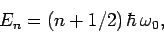(1135)

whereis the frequency of the corresponding classical oscillator. Here, the quantum number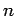takes the values. Let thebe the (real) properly normalized unperturbed eigenstates of the system.

Suppose that the electron is initially in an excited state: i.e.,. In principle, the electron can decay to a lower energy state via the spontaneous emission of a photon of the appropriate frequency. Let us investigate this effect. Now, according to Eq. (1131), the system can only make a spontaneous transition from an energy state corresponding to the quantum numberto one corresponding to the quantum number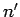if the associated electric dipole moment(1136)

is non-zero [since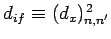for the case in hand]. However, according to Eq. (416),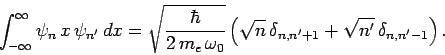(1137)

Since we are dealing with emission, we must have. Hence, we obtain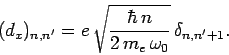(1138)

It is clear that (in the electric dipole approximation) we can only have spontaneous emission between states whose quantum numbers differ by unity. Thus, the frequency of the photon emitted when theth excited state decays is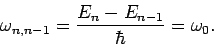(1139)

Hence, we conclude that, no matter which state decays, the emitted photon always has the same frequency as the classical oscillator.

According to Eq. (1131), the decay rate of theth excited state is given by(1140)

It follows that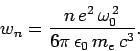(1141)

The mean radiated power is simply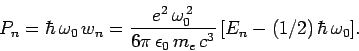(1142)

Classically, an electron in a one-dimensional oscillator potential radiates at the oscillation frequencywith the mean power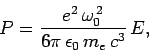(1143)

whereis the oscillator energy. It can be seen that a quantum oscillator radiates in an almost exactly analogous manner to the equivalent classical oscillator. The only difference is the factor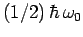in Eq. (1142)--this is needed to ensure that the ground-state of the quantum oscillator does not radiate.Next: Selection Rules Up: Time-Dependent Perturbation Theory Previous: Spontaneous Emission
Richard Fitzpatrick 2010-07-20